# A.4 Rotational velocityFigure A.8: Frame B rotating with respect to frame A.

Given two 3D coordinate frames A and B, the rotational, or angular, velocity of B with respect to A is given by a 3D vector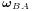(Figure A.8).is related to the derivative of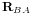by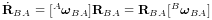(A.21)

where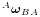and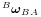indicatewith respect to frames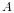and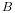and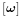denotes the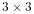cross product matrix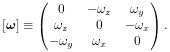(A.22)

If we consider instead the velocity ofwith respect to, it is straightforward to show that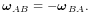(A.23)